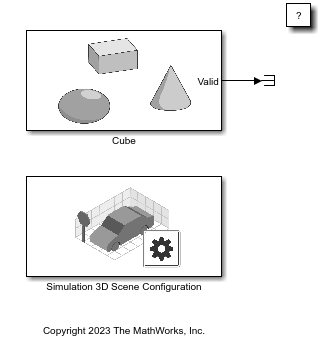# createMesh

Create new mesh with specified values

Since R2022b

## Syntax

``createMesh(actor,vertices,normals,faces)``
``createMesh(___,tcoords,vcolor)``

## Description

example

````createMesh(actor,vertices,normals,faces)` creates a mesh defined by `vertices`, `normals` and `faces`.```
````createMesh(___,tcoords,vcolor)` creates a mesh additionally defined by the texture coordinates `tcoords` and the vertex colors `vcolor`.```

## Examples

collapse all

This example shows how to build an actor from mesh data using the `createMesh` function and how to use the texture property of the `sim3d.Actor` object. First, you create a world scene and an actor object. Next, you build the appearance of the actor from a mesh and apply a texture. Then, you add the actor to the world, transform the actor, and set a view in the scene. Finally, you view the actor in the Simulation 3D Viewer window.

A mesh is a 3D build of a model consisting of polygons and is defined by vertices, normals, and faces. If you require detailed control over the geometry of an actor, create an actor from a mesh. This example uses sphere geometry for the mesh data to build the actor.

Build Actor from Mesh in World

Create a world scene.

`world = sim3d.World();`

Instantiate an actor object named `sphere`. Use the `sim3d.utils.Geometry` function to create a sphere. Obtain the sphere vertices `V`, normals `N`, faces `F`, texture coordinates `T`, and vertex colors `C`.

```ActObj = sim3d.Actor('ActorName', 'sphere'); [V, N, F, T, C] = sim3d.utils.Geometry.sphere([0.5 0.5 0.5]);```

Create a mesh using the actor object and sphere geometry. Set a texture provided by the image file. Add the actor object to the world.

```createMesh(ActObj, V, N, F, T, C); ActObj.Texture = fullfile(pwd,"image.png"); add(world,ActObj);```

Set Actor Transformation

Use the actor object translation, rotation, and scale properties to orient the actor relative to the world origin.

```ActObj.Translation = [0 0 0]; ActObj.Rotation = [0, 0, 0]; ActObj.Scale = [1, 1, 1];```

Set Viewer Window Point of View

If you do not create a viewport, then the point of view is set to 0, 0 ,0, and you can use the arrow keys and pointer to navigate in the Simulation 3D Viewer window.

For this example, use the `createViewport` function to create a viewport with a single field, `Main`, that contains a `sim3d.sensors.MainCamera` object.

```viewport = createViewport(world); viewport.Translation = [-3, 0, 0];```

Run Animation

Run a simulation set for 10 seconds with a sample time of 0.02 seconds.

`run(world,0.02,10)`Delete World

Delete the world object.

`delete(world)`

## Input Arguments

collapse all

Vertex positions, specified as a real positive (N,3) vector. This vector includes all vertex positions to be used for the mesh geometry.

Example: `vertices = reshape(1: 6, 2, 3)`

Data Types: `double`

Actor class where mesh is created, specified as a `sim3d.Actor` object.

Faces of actor shape, specified as a real positive (N,3) vector. This vector defines how each triangle of the mesh is drawn. Length must be a multiple of 3.

Example: `faces = [1: 3; 4 : 6]`

Data Types: `double`

Normal vectors for each vertex, specified as a real positive (N,3) vector. This vector must be the same length as `vertices` vector.

Example: `normals = reshape(7: 12, 2, 3)`

Data Types: `double`

Vertex colors, specified a real positive (N,3) vector. This vector must be the same length as `vertices` vector.

Example: `vertexcolor = reshape(25 : 33, 3, 3)`

Data Types: `double`

Texture coordinates of each vertex, specified a real positive (N,2) vector. This must be the same length as `vertices` array.

Example: `texturecoord = reshape(21 : 24, 2, 2)`

Data Types: `double`

## Version History

Introduced in R2022b